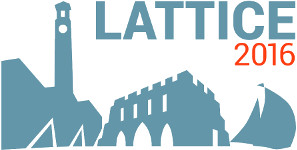#The 34th International Symposium on Lattice Field Theory (Lattice 2016)

Jul 24 – 30, 2016
Highfield Campus, University of Southampton
Europe/London timezone

## The leading order hadronic contribution of the anomalous magnetic moment of the muon with O$(a)$-improved Wilson fermions with Pade approximants from fits and time moments

Jul 26, 2016, 2:40 PM
20m
Building B2a Room 2077 (Highfield Campus, University of Southampton)

### Speaker

Hanno Horch (University of Mainz)

### Description

We present results of our lattice QCD study of the hadronic vacuum polarization (HVP) function with O$(a)$-improved $N_{\rm f}=2$ Wilson fermions with twisted boundary conditions. We discuss the extraction of the leading order hadronic contribution to the anomalous magnetic moment of the muon $\left(a_\mu^{\mathrm{HLO}}\right)$ via the hybrid method involving two steps: (i) To describe the low $Q^2$ range, we construct Pade approximants obtained either from correlated fits to the HVP or from time moments, which we obtain from derivatives of the current-current correlator.(ii) For the large $Q^2$ range we use a numerical integration. We estimate systematic uncertainties of the continuum and chiral extrapolations with the extended frequentist method. Preliminary results for $u,d,s$ and $c$ valence quarks at the physical point for $a_\mu^{\mathrm{HLO}}$ will be presented.

### Primary author

Hanno Horch (University of Mainz)

### Co-authors

Dr Andreas Nyffeler (University of Mainz) Dr Benjamin Jaeger (Swansea University) Dr Gregorio Herdoiza (Universidad Autónoma de Madrid) Prof. Hartmut Wittig (University of Mainz)

 Slides